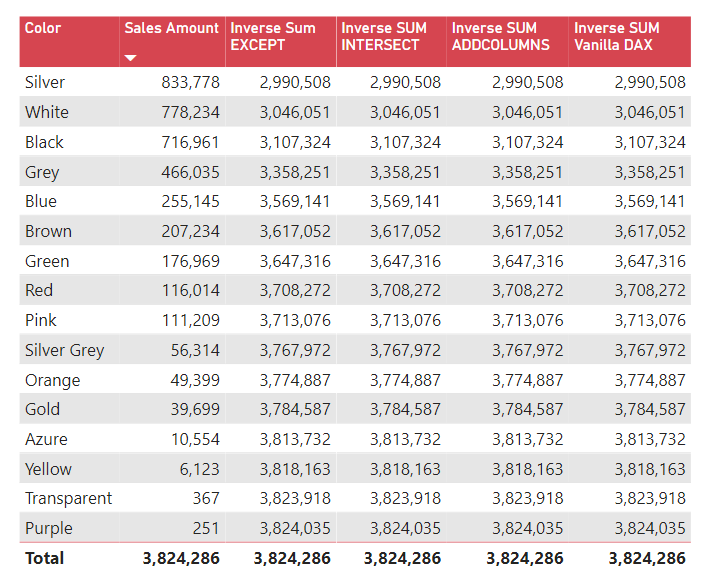Search
•Antriksh Sharma

# Inverse SUM in DAX

Updated: May 30

In this blog let's see how to compute value of the measure for every other value of a column except for the one that is currently visible in the current filter context.

Snapshot of the data model:The reporte we are starting with comprises of Products[Color] and Sales AmountSo taking example of Purple what we want to do is to show the sales of every color except Purple and similarly do the same calculation for each color, so the result at the Purple cell would be 3,824,286 ( Sales of all colors )- 251 ( Sales of Purple color )

Let's start creating the code of the measure step by step and I will show you multiple ways of doing such calculations, hence by the end of the blog you will have learnt different ways of approaching a problem.

## Using EXCEPT

EXCEPT is a DAX function that does SET operation and removes the rows of the second table from the table supplied in the first argument:The first thing is to create a new measure and then we need a function to retrieve the value of the product color that is visible in the filter context, and the function that wer are going to use is called SELECTEDVALUE, which simply retrieves the value of the column visible in the current filter context if only 1 value is visible otherwise it returns a BLANK

So using the below code we will get the value visible in the current filter context:

Next we need to remove the visible color from ALL colors that we have in the Products table and for that we are going to use another variable that utilizes the EXCEPT function

Notice how VisibleColor variable is wrapped inside curly braces? That's because curly braces are a shorthand for table constructors, EXCEPT is a table function and it only accepts table so we need to convert VisibleColor variable into a table that's why I have wrapped that inside curly braces

Now all we need to do is inject OtherColors variable into filter context using CALCULATE and then compute the total sales

Here is the complete code written so farNow to verify if this works you can simply subtract the sales amount of Purple from the grand total

Before checing other versions it is of paramount importance to clear that why VALUES can't be used here instead of SELECTEDVALUE, the issue is at the grand total there is no filter context, so VALUES will return all of the Products[Color] and when EXCEPT will remove the list of colors returned by VALUES from the list of colors returned by ALL, the result of EXCEPT won't contain any color and hence we would get BLANKThe above image shows what will happen if you use VALUES instead of SELECTEDVALUE

## Using INTERSECT

Since we have already seen a SET operator, I thought it would make sense to write about INTERSECT right after EXCEPT

Since the idea of what we are doing is clear, from here on to reduce verbosity of the blog I will squeeze everything into large steps

So as a curious person the first thing you would do is create a DAX code comprising of INTERSECT this way:

But the problem is it just returns the sales amount of the current product as shown in the below image:The problem is INTERSECT creates an intersection between let's say Purple and ALL Colors, and only returns a value when that value exists in both tables, which is basically like calculating Sales Amount but with a more complex measure.

To compute the Inverse SUM using INTERSECT we use the below code:

The above code stores the value of the All Sales in the TotalSales variable by removing any filter from the Products[Color] and then we check using ISINSCOPE if the Color column is being used for grouping, if yes, then we subtract the sales of the current product from the TotalSales else we simply return TotalSales (which is only returned at the grand total)

Comparing EXCEPT and INTERSECT side by side:ADDCOLUMNS, my most favourite function right after CALCULATE and CALCULATETABLE.

To compute inverse sum using ADDCOLUMNS we need to use the following code:

As explained above TotalSales variable computes the sales of ALL products color, i.e. the grand total then we check if the Color column is being using for grouping, if yes, then we prepare a temporary table in memory contains Color and the Sales amount, then we iterate that temporary table using SUMX and sum the [@Sales] column, and in the FALSE branch of IF we return the TotalSales variable which is required for the grand total.

Comparing all the measures created so far:## Vanilla DAX

Now for the simple example used above using ADDCOLUMNS, INTERSECT, or EXCEPT is proably an overkill but it never hurts to know more, right?

For the final example let's compute the same inverse sum using none of the above DAX functions:

Now the readers can deduce themselves how this simple measure works.

Comparing all 4 measures:## Recent Posts

See All

#### Tabular Editor 3 Course

TE3 is the ultimate development tool for creating Tabular Model and recently I got an opportunity to make a full fledged course on it for Enterprise DNA. Here is a post about that and how you can secu